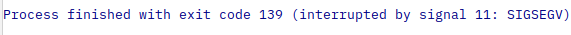# [Relay] How to insert parameter values? Compilation segfaults

Hi,

I’m trying to compile just a conv2d operator to test end-to-end compilation. I’ve made a separate conv2d strategy that tensorizes the incoming schedule. Compilation from Relay is done with this script:

``````# Relay high-level API compilation of Conv2D

import tvm
import tvm.relay as relay
import numpy as np

b = 2       # Input batch (also called N sometimes)
c = 3       # Input channels
x = 256     # Input width (also called W sometimes)
y = 256     # Input height (also called H sometimes)

fx = 5      # Filter width
fy = 5      # Filter height
k = 32      # Filter output channels

data_type = "int8"
act_shape = (b, c, y, x)
wgt_shape = (k, c, fx, fy)

activations = relay.var("act", tvm.relay.TensorType(act_shape, data_type))
weights = relay.var("wgt", tvm.relay.TensorType(wgt_shape, data_type))

# Only provide spatial dimension to kernel_size, otherwise, it doesn't work!
# Make padding "same" --> padding should be equal to fx // 2
conv = relay.nn.conv2d(activations, weights,kernel_size=(fx,fy), padding=fx // 2)

func = relay.Function(relay.analysis.free_vars(conv),conv)

print(func)

mod = tvm.ir.IRModule().from_expr(func)

random_weights = np.random.randint(0,5,size=wgt_shape).astype(data_type)
random_data = np.random.randint(0,5,size=act_shape).astype(data_type)

weights_params = tvm.nd.array(random_weights)
activations_params = tvm.nd.array(random_data)

params = {"act": random_data,
"wgt": random_weights}

with tvm.transform.PassContext(opt_level=3):
graph, lib, weights = relay.build(mod, target="c", params=params)

print(graph)
print(lib)
print(weights)

with open('/tmp/graph.json','w') as file:
file.write(graph)
with open('/tmp/weights.json','w') as file:
file.write(str(weights))

file_name = "conv2d.so"
lib.export_library(file_name, workspace_dir="/tmp")
``````

It works without problem when I don’t supply any parameters, but when I try to insert activations and weights, the compilation halts and the program segfaults.I think I’m not inserting the arrays correctly… Also maybe the relay graph I generate is not generated correctly, but I found it difficult to extract from the documentation to even just generate this simple example. Does anyone have help on this? I only managed to insert tensor values by using the graphruntime (`set_input`) but I don’t want to use that here, as I’m using a custom C backend (the normal C backend also segfaults).

Any suggestions? Thanks!